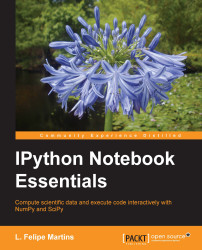•#### IPython Notebook Essentials#### Overview of this book

IPython Notebook EssentialsCreditswww.PacktPub.comPrefaceFree Chapter
A Tour of the IPython NotebookThe Notebook InterfaceGraphics with matplotlibHandling Data with pandasAdvanced Computing with SciPy, Numba, and NumbaProIPython Notebook Reference CardA Brief Review of PythonNumPy ArraysIndex## The Series class

A `Series` object represents a one-dimensional, indexed series of data. It can be thought of as a dictionary, with one main difference: the indexes in a `Series` class are ordered. The following example constructs a `Series` object and displays it:

```grades1 = Series([76, 82, 78, 100],
index = ['Alex', 'Robert', 'Minnie', 'Alice'],
name = 'Assignment 1', dtype=float64)
```

This produces the following output:

```Alex       76
Robert     82
Minnie     78
Alice     100
Name: Assignment 1, dtype: float64
```

Notice the format of the constructor call:

```Series(<data>, index=<indexes>, name=<name>, dtype=<type>)
```

Both `data` and `indexes` are usually lists or `NumPy` arrays, but can be any Python iterable. The lists must have the same length. The `name` variable is a string that describes the data in the series. The `type` variable is a `NumPy` data type. The `indexes` and the `name` variables are optional (if `indexes` are omitted, they are set to integers...